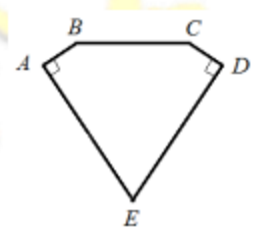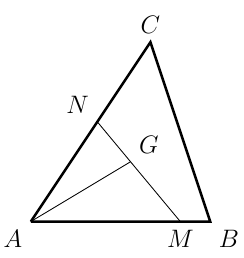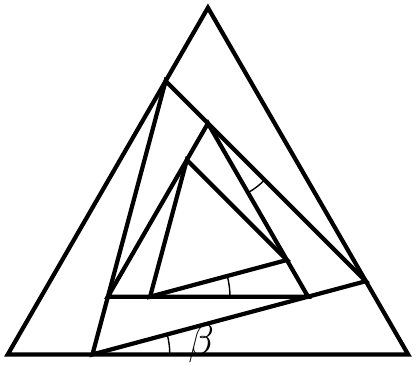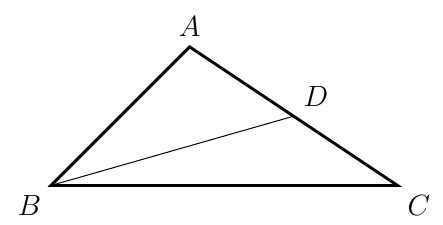#### China

###### back to index

If the middle term of three consecutive integers is a perfect square, then the product of these three numbers is called a $\textit{beautiful}$ number. What is the greatest common divisor of all the $\textit{beautiful}$ numbers?

Find the largest 7-digit integer such that all its 3-digit subpart is either a multiple of 11 or multiple of 13.

Find a $4$-digit square number $x$ such that if every digit of $x$ is increased by 1, the new number is still a perfect square.

If $a+b=\sqrt{5}$, compute $\frac{a^2 -a^2b^2 + b^2 +2ab}{a+ab+b}$.

What is the units digit of $-1\times 2008 + 2 \times 2007 - 3\times 2006 + 4\times 2005 +\cdots-1003\times 1006 + 1004 \times 1005$?

If $-1 < a < b < 0$, then which relationship below holds? $(A)\quad a < a^3 < ab^2 < ab \qquad (B)\quad a < ab^2 < ab < a^3 \qquad (C)\quad a< ab < ab^2 < a^3 \qquad (D) a^3 < ab^2 < a < ab$

Let point $A$ and $B$ represent real numbers $a$ and $b$, respectively. If $A$ and $B$ lay on different sides of the origin $O$, and $|a - b| = 2016$, $AO = 2BO$, what is the value of $a+b$?

If $a+b=\sqrt{5}$, compute the value of $\frac{a^2 - a^2b^2 + b^2 +2ab}{a+ab+b}+ab$.

If $AE=DE=5$, $AB=CD$, $BC=4$, $\angle{E}=60^\circ$, $\angle{A}=\angle{D}=90^\circ$, then what is the area of the pentagon $ABCDE$?How many solutions does the following system have? $$\left\{ \begin{array}{ll} \lfloor x \rfloor + 2y &= 1\\ \lfloor y \rfloor + x &=2 \end{array} \right.$$ Where $\lfloor x \rfloor$ and $\lfloor y \rfloor$ denote the largest integers not exceeding $x$ and $y$, respectively.

Let $X$ be the integer part of $\left(3+\sqrt{7}\right)^n$ where $n$ is a positive integer. Show that $X$ must be odd.

Is it possible to arrange these numbers, $1, 1, 2, 2, 3, 3, \cdots, 1986, 1986$ to form a sequence for such there is $1$ number between two $1$'s, $2$ numbers between two $2$'s, $\cdots$, $1986$ numbers between two 1986's?

Let even function $f(x)$ and odd function $g(x)$ satisfy the relationship of $f(x)+g(x)=\sqrt{1+x+x^2}$. Find $f(3)$.

Let $f\Big(\dfrac{1}{x}\Big)=\dfrac{1}{x^2+1}$. Compute $$f\Big(\dfrac{1}{2013}\Big)+f\Big(\dfrac{1}{2012}\Big)+f\Big(\dfrac{1}{2011}\Big)+\cdots +f\Big(\dfrac{1}{2}\Big)+f(1)+f(2)+\cdots +f(2011)+f(2012)+f(2013)$$

Let $f(x)=x^{-\frac{k^2}{2}+\frac{3}{2}k+1}$ be an odd function where $k$ is an integer. If $f(x)$ is monotonically increasing when $x\in(0,+\infty)$, find all the possible values of $k$.

Let $G$ be the centroid of $\triangle{ABC}$. Points $M$ and $N$ are on side $AB$ and $AC$, respectively such that $\overline{AM} = m\cdot\overline{AB}$ and $\overline{AN} = n\cdot\overline{AC}$ where $m$ and $n$ are two positive real numbers. Find the minimal value of $mn$.An infinite number of equilateral triangles are constructed as shown on the right. Each inner triangle is inscribed in its immediate outsider and is shifted by a constant angle $\beta$. If the area of the biggest triangle equals to the sum of areas of all the other triangles, find the value of $\beta$ in terms of degrees.Let $BD$ be a median in $\triangle{ABC}$. If $AB=\frac{4\sqrt{6}}{3}$, $\cos{B}=\dfrac{\sqrt{6}}{6}$, and $BD=\sqrt{5}$, find the length of $BC$ and the value of $\sin{A}$.Suppose function $f(x)=\frac{1+x}{1-x}$. Evaluate $$f\Big(\frac{1}{2}\Big)\cdot f\Big(\frac{1}{4}\Big)\cdot f\Big(\frac{1}{6}\Big)\cdots f\Big(\frac{1}{2014}\Big)$$

Let the sum of first $n$ terms of arithmetic sequence $\{a_n\}$ be $S_n$, and the sum of first $n$ terms of arithmetic sequence $\{b_n\}$ be $T_n$. If $\frac{S_n}{T_n}=\frac{2n}{3n+7}$, compute the value of $\frac{a_8}{b_6}$.

Suppose every term in the sequence $$1, 2, 1, 2, 2, 2, 1, 2, 2, 2, 2, 2, 1, \cdots$$ is either $1$ or $2$. If there are exactly $(2k-1)$ twos between the $k^{th}$ one and the $(k+1)^{th}$ one, find the sum of its first $2014$ terms.

Let $c_1, c_2, c_3, \cdots$ be a series of concentric circles whose radii form a geometric sequence with common ratio as $r$. Suppose the areas of rings which are formed by two adjacent circles are $S_1, S_2, S_3, \cdots$. Which statement below is correct regarding the sequence $\{S_n\}$? A) It is not a geometric sequence B) It is a geometric sequence and its common ratio is $r$ C) It is a geometric sequence and its common ratio is $r^2$ D) It is a geometric sequence and its common ratio is $r^2-1$

Given the sequence $\{a_n\}$ satisfies $a_n+a_m=a_{n+m}$ for any positive integers $n$ and $m$. Suppose $a_1=\frac{1}{2013}$. Find the sum of its first $2013$ terms.

If real number $x$ satisfies $x^4 - 2x^3 -7x^2 + 8x +12\le 0$, find the max value of $|x+\frac{4}{x}|$

Find the range of function $f(x)=3^{-|\log_2x|}-4|x-1|$.